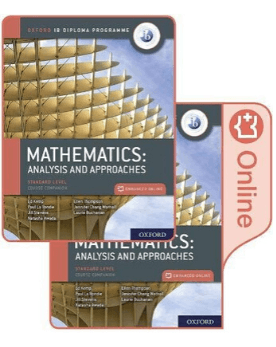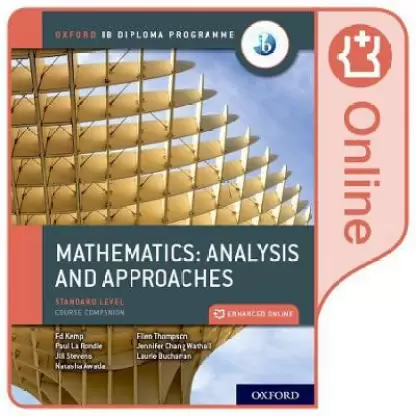# IB Mathematics: analysis and approaches, SL Course Companion

translation missing: en.products.product.price.regular_price \$115.00
translation missing: en.products.product.price.sale_price \$115.00 translation missing: en.products.product.price.regular_price
Featuring a wealth of digital content, this concept-based Print and Enhanced Online Course Book Pack has been developed in cooperation with the IB to provide the most comprehensive support for the new DP Mathematics: analysis and approaches SL syllabus, for first teaching in September 2019.

### Features

• Address all aspects of the new DP Mathematics: analysis and approaches SL syllabus via an Enhanced Online Course Book Pack - made up of one full-colour, print textbook and one online textbook, including extensive teacher notes
• Ensure learners are ready to tackle each topic with targeted 'Prior Knowledge' worksheets, linked to 'Before You Start' summaries and exercises at the start of every chapter
• Deliver in-depth coverage of all topics through clear explanations and worked solutions, animated worked examples, differentiated exercises and worksheets, with answers provided
• Adopt a concept-based approach with conceptual lenses and microconcepts woven into every chapter, plus rich investigations that integrate factual and conceptual questions - leading to meaningful, content-specific conceptual understanding
• Deepen mathematical understanding via inquiry-based tasks that relate to the content of each chapter, 'international mindedness' features, regular links to Theory of Knowledge, and activities that target ATL skills
• Support students' development of a mathematical toolkit, as required by the new syllabus, with modelling and investigation activities presented in each chapter, including prompts for reflection, and suggestions for further study
• Thoroughly prepare students for IB assessment via in-depth coverage of course content, overviews of all requirements, exam-style practice questions and papers, and a full chapter supporting the new mathematical exploration (IA)
• Includes support for the most popular Graphic Display Calculator models
• This Online Course Book will be available on Oxford Education Bookshelf until 2029. Access is facilitated via a unique code, which is sent in the mail. The code must be linked to an email address, creating a user account.
al user.

From patterns to generalizations: sequences and series
1.1: Number patterns and sigma notation
1.2: Arithmetic and geometric sequences
1.3: Arithmetic and geometric series
1.4: Modelling using arithmetic and geometric series
1.5: The binomial theorem
1.6: Proofs
Representing relationships: introducing functions
2.1: What is a function?
2.2: Functional notation
2.3: Drawing graphs of functions
2.4: The domain and range of a function
2.5: Composition of functions
2.6: Inverse functions
Modelling relationships: linear and quadratic functions
3.1: Parameters of a linear function
3.2: Linear functions
3.3: Transformations of functions
3.5: Solving quadratic equations by factorization and completing the square
3.6: The quadratic formula and the discriminant
Equivalent representations: rational functions
4.1: The reciprocal function
4.2: Transforming the reciprocal function
4.3: Rational functions of the form ax+b/cx+d
Measuring change: differentiation
5.1: Limits and convergence
5.2: The derivative function
5.3: Differentiation rules
5.4: Graphical interpretation of first and second derivatives
5.5: Application of differential calculus: optimization and kinematics
Representing data: statistics for univariate data
6.1: Sampling
6.2: Presentation of data
6.3: Measures of central tendency
6.4: Measures of dispersion
Modelling relationships between two data sets: statistics for bivariate data
7.1: Scatter diagrams

7.2: Measuring correlation
7.3: The line of best fit
7.4: Least squares regression
Quantifying randomness: probability
8.1: Theoretical and experimental probability
8.2: Representing probabilities: Venn diagrams and sample spaces
8.3: Independent and dependent events and conditional probability
8.4: Probability tree diagrams
Representing equivalent quantities: exponentials and logarithms
9.1: Exponents
9.2: Logarithms
9.3: Derivatives of exponential functions and the natural logarithmic function
From approximation to generalization: integration
10.1: Antiderivatives and the indefinite integral
10.2: More on indefinite integrals
10.3: Area and definite integrals
10.4: Fundamental theorem of calculus
10.5: Area between two curves
Relationships in space: geometry and trigonometry in 2D and 3D
11.1: The geometry of 3D shapes
11.1: Right-angles triangle trigonometry
11.3: The sine rule
11.4: The cosine rule
11.5: Applications of right and non-right angled trigonometry
Periodic relationships: trigonometric functions
12.1: Radian measure, arcs, sectors and segments
12.2: Trigonometric ratios in the unit circle
12.3: Trigonometric identities and equations
12.4: Trigonometric functions
Modelling change: more calculus
13.1: Derivatives with sine and cosine
13.2: Applications of derivatives
13,3: Integration with sine, cosine and substitution
13.4: Kinematics and accumulating change
Valid comparisons and informed decisions: probability distributions
14.1: Random variables
14.2: The binomial distribution
14.3: The normal distribution
Exploration

Author: Paul La Rondie, Jill Stevens, Natasha Awada, Jennifer Chang Wathall

Curriculum: IB Diploma Programme International Baccalaureate

Dimension: 10.1 x 1.1 x 7.7 inches

Format: Paperback with Printed Access Code

Isbn 10: 0198427107

Language: English

Pages: 672 pages

Release date: 04/15/2019

Series: Oxford IB Diploma Programme: IB Mathematics

Year: 2019